Ch 7. Incompressible and Inviscid Flow Multimedia Engineering Fluids Bernoulli'sEquation FlowMeasurements Superpositionof Flows Flow aroundCylinder
 Chapter 1. Basics 2. Fluid Statics 3. Kinematics 4. Laws (Integral) 5. Laws (Diff.) 6. Modeling/Similitude 7. Inviscid 8. Viscous 9. External Flow 10. Open-Channel Appendix Basic Math Units Basic Equations Water/Air Tables Sections Search eBooks Dynamics Fluids Math Mechanics Statics Thermodynamics Author(s): Chean Chin Ngo Kurt Gramoll ©Kurt GramollFLUID MECHANICS - CASE STUDY SOLUTION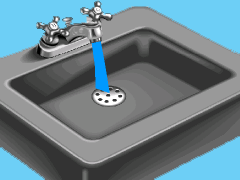Problem Diagram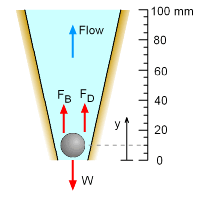Rotameter The flow rate of the faucet is given by Q = umAm The float of the rotameter is 10 mm from its base (i.e., y =10 mm). Hence, the area of the annular space is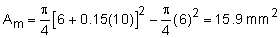The mean velocity of the water passing through the annular space is given by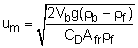The volume and frontal area of the ball are calculated to be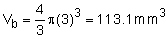Now, the mean velocity um can be calculated as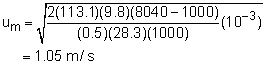The volume flow rate is thus given by      Q = (1.05 m/s)(15.9 mm2) = 16.7 (10-6) m3/s The total volume of the water wasted in a 30-day period is       Vtotal = (16.7)(10-6)(30)(24)(60)(60) = 43.3 m3 The corresponding cost is    = (43.3 m3) (\$1.75/1000 gal) (1 gal/0.003785 m3)    = \$20.02

Practice Homework and Test problems now available in the 'Eng Fluids' mobile app
Includes over 250 problems with complete detailed solutions.
Available now at the Google Play Store and Apple App Store.